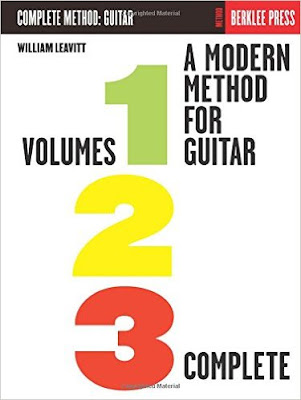# Berklee Guitar練習計畫畢業前我在西門町的九五樂府找到了一本Berklee出版的吉他教材，叫做A Modern Method For Guitar,Volume 1,2,3 Complete，書名非常的酷炫，雖然要價一千多但我還是買了下來，回到家中打開才發現天啊，內容超級無敵難，因為裡面完全沒有吉他專用的六線譜，只有一般的五線譜，翻了一頁我就把它丟到書架上冰起來了……
直到最近比較有空，又覺得練曲子練得有點無聊，是時候要來加強基本功了，又把本書翻出來開始練，為了讓練習不留白，想把練習的紀錄記在網誌上，也算是對自己有個交代，也希望可以幫助到同樣擁有這本書的朋友們。

#### 以下連結到對應的文章

Volume1
Lesson 4: Note and Chord Review (Upper Register)(P.8)
Lesson 5: One,Two,Three,Four (Duet)(P.10)
Lesson 6: Rhythm Accompaniment(P.11)
Lesson 7: Review (Lower Register)(P.13)
Lesson 8: Imitation Duet (P.14)
Lesson 9: Sharps and Flats (P.15)
Lesson 10: Here We Go Again (duet)(P.16)

Lesson 11: Rhythm Accompaniment(Base Notes and Chords)(P.17)
Lesson 12: Eighth Notes- Counting and Picking (P.18)
Lesson 13: Etude No.1(Duet) (P.20)
Lesson 14: Rests, Tied Notes, Dotted Notes (P.21)
Lesson 15: Etude No.2 (Duet) (P.22)
Lesson 16: First Solo (P.22)
Lesson 17: Rhythm Accompaniment (Chord Diagram Explained)(P.24)
Lesson 18: Second Solo (P.25)
Lesson 19: Etude No.3 (Duet) (P.25)
Lesson 20: Picking Etude No.1 (Right-Hand Development) (P.27)

Lesson 21: Etude (P.27)
Lesson 22: Two, Two (duet) (P.28)
Lesson 23: Key of G Major (First position) (P.30)
Lesson 24: Rhythm Accompaniment(Chord Diagram) (P.30)
Lesson 25: Sixteen Notes (P.31)
Lesson 26: Duet in G (P.32)
Lesson 27: Picking Etude No.2 (P.32)
Lesson 28: Another Duet in G (P.34)
Lesson 29: Key of F major (First Position) (P.35)
Lesson 30: Rhythm Accompaniment (Chord Diagrams and Grand Barre)(P.35)

Lesson 31: Duet in F (P.36)
Lesson 32: The Triplet (P.37)
Lesson 33: Waltz in F (Solo) (P.38)
Lesson 34: Key of A Minor (First Position) (P.39)
Lesson 35: Rhythm Accompaniment (Chord Diagrams)(P.39)
Lesson 36: Pretty Pickin'(Duet)(P.40)
Lesson 37: Dotted Eighth and Sixteenth Notes (P.42)
Lesson 38: Key of E Minor (Scales in First Position)(P.43)
Lesson 39: Rhythm Accompaniment(Chord Diagrams)(P.43)

Lesson 41: Rhythm Accompaniment(Movable Chord Forms)(P.45)
Lesson 42: Chromatic Scales(First Position)(P.46)
Lesson 43: Speed Studies(P.46)
Lesson 44: Key of D Minor (Scales in First Position)(P.47)
Lesson 45: Rhythm Accompaniment(P.47)
Lesson 46: Endurance Etude(Picking Etude No.3)(P.48)
Lesson 47: Key of Bb(Scales in First Position)(P.50)
Lesson 48: Rhythm Accompaniment(P.50)
Lesson 49: Duet in Bb(P.51)
Lesson 50: Reverse Alternate Picking Study(P.52)

Lesson 51: Key of D Major(Scale in First Position)(P.53)
Lesson 52: Duet in D(P.53)
Lesson 53: Dynamic Etude- Etude No.4(duet)(P.54)
Lesson 54: Key of A Major (First Position)(P.56)
Lesson 55: Duet in A(P.56)
Lesson 56: Rhythm Accompaniment(P.56)
Lesson 57: Key of Eb Major(Scale in First Position)(P.57)
Lesson 58: Duet in Eb(P.57)
Lesson 59: Movable Chord Forms(P.58)
Lesson 60: Chord Simplification and Substitution Chart(P.58)

Lesson 61: Picking – A Different Technique(P.59)
Lesson 62: Position Playing(P.60)
Lesson 63: Major Scales(C Major, Fingering Type 1, Second Position)(P.60)
Lesson 64: Chord Etude No.1(P.62)
Lesson 65: Etude No.5 (P.63)

### 在〈Berklee Guitar練習計畫〉中有 1 則留言

1.Unknown表示:

我之前也在来练习这个，练到SEA TO SEA 就没练了，要上班，在公司都要被虐成狗了，下班就想躺尸， 一定要找个时间接着把他练完，你的练习视频也一定要继续拍下去啊，会来看你哦